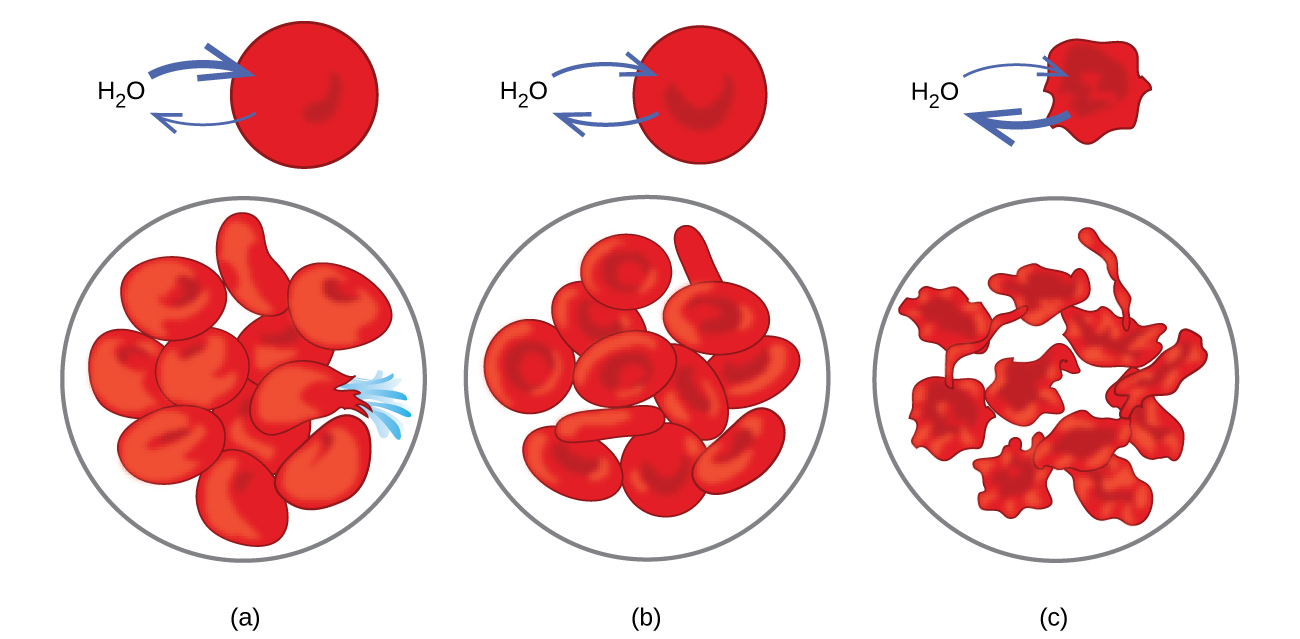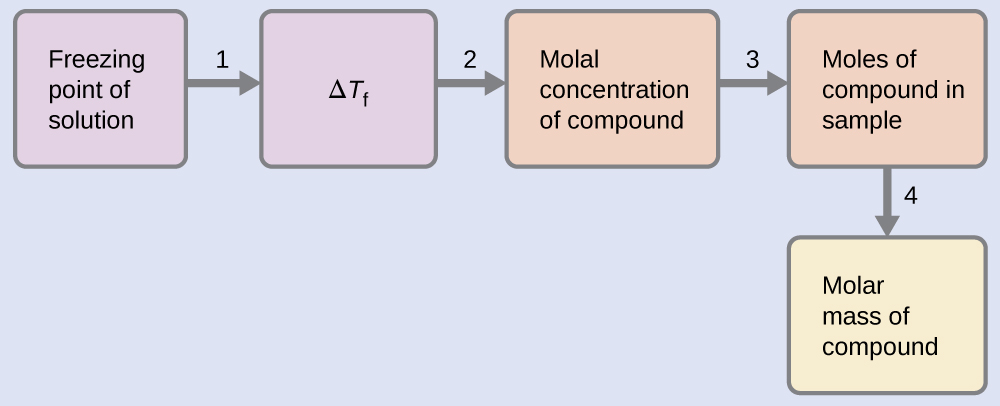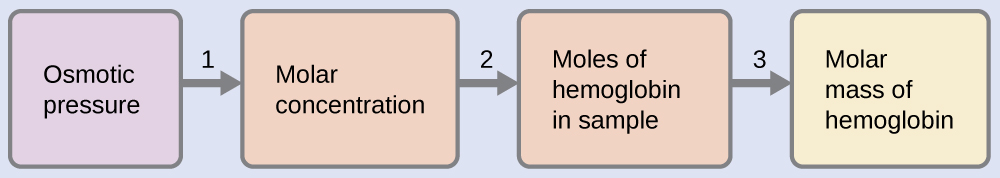11.4 Colligative properties  (Page 8/30)

 Page 8 / 30Red blood cell membranes are water permeable and will (a) swell and possibly rupture in a hypotonic solution; (b) maintain normal volume and shape in an isotonic solution; and (c) shrivel and possibly die in a hypertonic solution. (credit a/b/c: modifications of work by “LadyofHats”/Wikimedia commons)

Determination of molar masses

Osmotic pressure and changes in freezing point, boiling point, and vapor pressure are directly proportional to the concentration of solute present. Consequently, we can use a measurement of one of these properties to determine the molar mass of the solute from the measurements.

Determination of a molar mass from a freezing point depression

A solution of 4.00 g of a nonelectrolyte dissolved in 55.0 g of benzene is found to freeze at 2.32 °C. What is the molar mass of this compound?

Solution

We can solve this problem using the following steps.1. Determine the change in freezing point from the observed freezing point and the freezing point of pure benzene ( [link] ).
$\text{Δ}{T}_{\text{f}}=5.5\phantom{\rule{0.2em}{0ex}}\text{°}\text{C}-2.32\phantom{\rule{0.2em}{0ex}}\text{°}\text{C}=3.2\phantom{\rule{0.2em}{0ex}}\text{°}\text{C}$
2. Determine the molal concentration from K f , the freezing point depression constant for benzene ( [link] ), and Δ T f .
$\begin{array}{l}\hfill \text{Δ}{T}_{\text{f}}={K}_{\text{f}}m\hfill \\ \\ m\phantom{\rule{0.2em}{0ex}}=\phantom{\rule{0.2em}{0ex}}\frac{\text{Δ}{T}_{\text{f}}}{{K}_{\text{f}}}\phantom{\rule{0.2em}{0ex}}=\phantom{\rule{0.2em}{0ex}}\frac{3.2\phantom{\rule{0.2em}{0ex}}\text{°}\text{C}}{5.12\phantom{\rule{0.2em}{0ex}}\text{°}\text{C}\phantom{\rule{0.2em}{0ex}}{m}^{-1}}\phantom{\rule{0.2em}{0ex}}=\phantom{\rule{0.2em}{0ex}}0.63\phantom{\rule{0.2em}{0ex}}m\end{array}$
3. Determine the number of moles of compound in the solution from the molal concentration and the mass of solvent used to make the solution.
$\text{Moles of solute}\phantom{\rule{0.2em}{0ex}}=\phantom{\rule{0.2em}{0ex}}\frac{0.62\phantom{\rule{0.2em}{0ex}}\text{mol solute}}{1.00\phantom{\rule{0.2em}{0ex}}\overline{)\text{kg solvent}}}\phantom{\rule{0.2em}{0ex}}×\phantom{\rule{0.2em}{0ex}}0.0550\phantom{\rule{0.2em}{0ex}}\overline{)\text{kg solvent}}=0.035\phantom{\rule{0.2em}{0ex}}\text{mol}$
4. Determine the molar mass from the mass of the solute and the number of moles in that mass.
$\text{Molar mass}\phantom{\rule{0.2em}{0ex}}=\phantom{\rule{0.2em}{0ex}}\frac{4.00\phantom{\rule{0.2em}{0ex}}\text{g}}{0.034\phantom{\rule{0.2em}{0ex}}\text{mol}}\phantom{\rule{0.2em}{0ex}}=\phantom{\rule{0.2em}{0ex}}1.2\phantom{\rule{0.2em}{0ex}}×\phantom{\rule{0.2em}{0ex}}{10}^{2}\text{g/mol}$

A solution of 35.7 g of a nonelectrolyte in 220.0 g of chloroform has a boiling point of 64.5 °C. What is the molar mass of this compound?

1.8 $×$ 10 2 g/mol

Determination of a molar mass from osmotic pressure

A 0.500 L sample of an aqueous solution containing 10.0 g of hemoglobin has an osmotic pressure of 5.9 torr at 22 °C. What is the molar mass of hemoglobin?

Solution

Here is one set of steps that can be used to solve the problem:1. Convert the osmotic pressure to atmospheres, then determine the molar concentration from the osmotic pressure.
$\begin{array}{}\\ \Pi \phantom{\rule{0.2em}{0ex}}=\phantom{\rule{0.2em}{0ex}}\frac{5.9\phantom{\rule{0.2em}{0ex}}\text{torr}\phantom{\rule{0.2em}{0ex}}×\phantom{\rule{0.2em}{0ex}}1\phantom{\rule{0.2em}{0ex}}\text{atm}}{760\phantom{\rule{0.2em}{0ex}}\text{torr}}\phantom{\rule{0.2em}{0ex}}=\phantom{\rule{0.2em}{0ex}}7.8\phantom{\rule{0.2em}{0ex}}×\phantom{\rule{0.2em}{0ex}}{10}^{-3}\text{atm}\\ \Pi =\mathit{\text{MRT}}\\ \\ M\phantom{\rule{0.2em}{0ex}}=\phantom{\rule{0.2em}{0ex}}\frac{\Pi }{RT}\phantom{\rule{0.2em}{0ex}}=\phantom{\rule{0.2em}{0ex}}\frac{7.8\phantom{\rule{0.2em}{0ex}}×\phantom{\rule{0.2em}{0ex}}{10}^{-3}\phantom{\rule{0.2em}{0ex}}\text{atm}}{\left(0.08206\phantom{\rule{0.2em}{0ex}}\text{L atm/mol K}\right)\left(295\phantom{\rule{0.2em}{0ex}}\text{K}\right)}\phantom{\rule{0.2em}{0ex}}=\phantom{\rule{0.2em}{0ex}}3.2\phantom{\rule{0.2em}{0ex}}×\phantom{\rule{0.2em}{0ex}}{10}^{-4}\text{M}\end{array}$
2. Determine the number of moles of hemoglobin in the solution from the concentration and the volume of the solution.
$\text{moles of hemoglobin}\phantom{\rule{0.2em}{0ex}}=\phantom{\rule{0.2em}{0ex}}\frac{3.2\phantom{\rule{0.2em}{0ex}}×\phantom{\rule{0.2em}{0ex}}{10}^{-4}\text{mol}}{1\phantom{\rule{0.2em}{0ex}}\overline{)\text{L solution}}}\phantom{\rule{0.2em}{0ex}}×\phantom{\rule{0.2em}{0ex}}0.500\phantom{\rule{0.2em}{0ex}}\overline{)\text{L solution}}=1.6\phantom{\rule{0.2em}{0ex}}×\phantom{\rule{0.2em}{0ex}}{10}^{-4}\text{mol}$
3. Determine the molar mass from the mass of hemoglobin and the number of moles in that mass.
$\text{molar mass}\phantom{\rule{0.2em}{0ex}}=\phantom{\rule{0.2em}{0ex}}\frac{10.0\phantom{\rule{0.2em}{0ex}}\text{g}}{1.6\phantom{\rule{0.2em}{0ex}}×\phantom{\rule{0.2em}{0ex}}{10}^{-4}\text{mol}}\phantom{\rule{0.2em}{0ex}}=\phantom{\rule{0.2em}{0ex}}6.2\phantom{\rule{0.2em}{0ex}}×\phantom{\rule{0.2em}{0ex}}{10}^{4}\text{g/mol}$

What is the molar mass of a protein if a solution of 0.02 g of the protein in 25.0 mL of solution has an osmotic pressure of 0.56 torr at 25 °C?

2.7 $×$ 10 4 g/mol

Colligative properties of electrolytes

As noted previously in this module, the colligative properties of a solution depend only on the number, not on the kind, of solute species dissolved. For example, 1 mole of any nonelectrolyte dissolved in 1 kilogram of solvent produces the same lowering of the freezing point as does 1 mole of any other nonelectrolyte. However, 1 mole of sodium chloride (an electrolyte) forms 2 moles of ions when dissolved in solution. Each individual ion produces the same effect on the freezing point as a single molecule does.

What is stoichometry
what is atom
An indivisible part of an element
ngwuebo
the smallest particle of an element which is indivisible is called an atom
Aloaye
An atom is the smallest indivisible particle of an element that can take part in chemical reaction
Alieu
is carbonates soluble
what is the difference between light and electricity
What is atom? atom can be defined as the smallest particles
what is the difference between Anode and nodes?
What's the net equations for the three steps of dissociation of phosphoric acid?
what is chemistry
the study of matter
Reginald
what did the first law of thermodynamics say
energy can neither be created or distroyed it can only be transferred or converted from one form to another
Graham's law of Diffusion
what is melting vaporization
melting and boiling point explain in term of molecular motion and Brownian movement
Anieke
Scientific notation for 150.9433962
what is aromaticity
aromaticity is a conjugated pi system specific to organic rings like benzene, which have an odd number of electron pairs within the system that allows for exceptional molecular stability
Pookieman
what is caustic soda
sodium hydroxide (NaOH)
Kamaluddeen
what is distilled water
Rihanat
is simply means a condensed water vapour
Kamaluddeen
a hydrocarbon contains 7.7 percent by mass of hydrogen and 92.3 percent by mass of carbon
how many types of covalent r there
how many covalent bond r there
JArim
they are three 3
TYPES OF COVALENT BOND-POLAR BOND-NON POLAR BOND-DOUBLE BOND-TRIPPLE BOND. There are three types of covalent bond depending upon the number of shared electron pairs. A covalent bond formed by the mutual sharing of one electron pair between two atoms is called a "Single Covalent bond.
UsmanByByBy Prateek AshtikarByBy